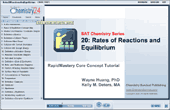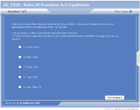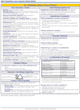How to Learn in 24 Hours?

 Need Help? M-F: 9am-5pm(PST): Toll-Free: (877) RAPID-10 or 1-877-727-4310 24/7 Online Technical Support: The Rapid Support Center vip@rapidlearningcenter.com Secure Online Order:Need Proof? Testimonials by Our Users

 Rapid Learning Courses: MCAT in 24 Hours (2021-22) USMLE in 24 Hours (Boards) Chemistry in 24 Hours Biology in 24 Hours Physics in 24 Hours Mathematics in 24 Hours Psychology in 24 Hours SAT in 24 Hours ACT in 24 Hours AP in 24 Hours CLEP in 24 Hours DAT in 24 Hours (Dental) OAT in 24 Hours (Optometry) PCAT in 24 Hours (Pharmacy) Nursing Entrance Exams Certification in 24 Hours eBook - Survival Kits Audiobooks (MP3)

 Tell-A-Friend: Have friends taking science and math courses too? Tell them about our rapid learning system.Home »  Chemistry »  SAT Chemistry

Reaction Rates & Equilibrium

 Topic Review on "Title": Kinetics is the study of the rate of reaction and the factors that affect it. Collision theory In order for a reaction to occur, the molecules must collide in the correct orientation with the minimum energy needed for the transition from the reactants to the products (the activation energy).  Only a very few collisions meet these requirements and result in a reaction. Reaction Coordinate Diagrams Reaction coordinate diagrams show the energy of the reactants, the activation energy up to the activated complex, or transition state (the in-between state between the reactants and the products), and the energy of the products.  The overall energy change of the reaction is also shown.  Factors affecting rate Increasing the temperature increases the number of collisions, and also the number of collisions with the needed energy.  Therefore, increasing temperature increases the rate of reaction.  Increasing the concentration or the surface area also increases the number of collisions, therefore increasing the chance that a successful collision will occur—which increases rate.  Adding a catalyst, a species that increases the rate of reaction without being used up in the reaction, also increases the rate. Equilibrium Dynamic equilibrium is when the rate of the forward and reverse reactions are equal.  The reaction will appear to have stopped, as the concentrations of each species won’t change, but the reaction continues to proceed in both directions. Equilibrium constants Equilibrium constant expressions are the ratio of the concentrations of products to reactants using their coefficients from the balanced equation as exponents.  Pure solids and liquids are not included in the equilibrium constant expression.  Equilibrium concentrations are plugged into the expression to solve for the equilibrium constant.  A large equilibrium constant means that the reaction “lies to the right” with more products than reactants at equilibrium.  A small constant means that the reaction “lies to the left” with more reactants the products present at equilibrium. Reaction Quotients The reaction quotient is the value when concentrations at any time are plugged into the equilibrium constant expression.  If the reaction quotient equals the equilibrium constant, then the system is at equilibrium.  If the reaction quotient is too large, the reaction will proceed to the left to produce more reactants (and reduce products).  The opposite will happen if the reaction quotient is too small. Le Chatelier’s Principle Le Chatelier’s principle says that if a system at equilibrium is stressed or changed, the system will shift to reach equilibrium again.  Whatever you do to the system, it will un-do it.  For example, if you add more reactants, the reaction will proceed to the right to get rid of the extra reactants and make more products.

Rapid Study Kit for "Title":
 Flash Movie Flash Game Flash Card Core Concept Tutorial Problem Solving Drill Review Cheat Sheet"Title" Tutorial Summary : Kinetics is the study of reaction rates.  This tutorial introduces factors affecting the rate of reaction.  Equilibrium is established when the rate of the forward reaction is equal to the rate of reaction of the reverse reaction in a reversible reaction.  This tutorial introduces how equilibrium is established, how equilibrium constant expressions are written and how equilibrium constants are calculated.  Le Chatelier’s principle will be introduced.

 Tutorial Features: Specific Tutorial Features: Animation of conditions necessary for a reaction to occur Simultaneous animation of a reaction and a reaction coordinate diagram Molecular animations of elementary steps in a reaction mechanism Molecular animation of establishment of dynamic equilibrium Animation of equations to show a change in the ratio of product to reactants Series Features: Concept map showing inter-connections of new concepts in this tutorial and those previously introduced. Definition slides introduce terms as they are needed. Visual representation of concepts Animated examples—worked out step by step A concise summary is given at the conclusion of the tutorial.

 "Title" Topic List: Collision Theory Reaction Coordinate Diagrams Factors affecting rate Dynamic equilibrium Equilibrium constant Writing expressions Calculating constants Meaning of the equilibrium constant Reaction quotient Writing expressions and finding the value Using it to determine if it’s at equilibrium Le Chatelier’s principle

See all 24 lessons in college chemistry, including concept tutorials, problem drills and cheat sheets:
Teach Yourself SAT Chemistry Visually in 24 Hours